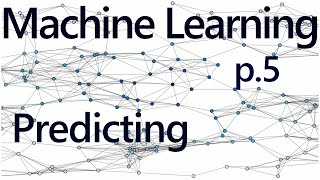# Regression forecasting and predicting - Practical Machine Learning Tutorial with Python p.5

In this video, make sure you define the X's like so. I flipped the last two lines by mistake:

X = np.array(df.drop(['label'],1))
X = preprocessing.scale(X)
X_lately = X[-forecast_out:]
X = X[:-forecast_out:]

To forecast out, we need some data. We decided that we're forecasting out 10% of the d...
In this video, make sure you define the X's like so. I flipped the last two lines by mistake:

X = np.array(df.drop(['label'],1))
X = preprocessing.scale(X)
X_lately = X[-forecast_out:]
X = X[:-forecast_out:]

To forecast out, we need some data. We decided that we're forecasting out 10% of the data, thus we will want to, or at least *can* generate forecasts for each of the final 10% of the dataset. So when can we do this? When would we identify that data? We could call it now, but consider the data we're trying to forecast is not scaled like the training data was. Okay, so then what? Do we just do preprocessing.scale() against the last 10%? The scale method scales based on all of the known data that is fed into it. Ideally, you would scale both the training, testing, AND forecast/predicting data all together. Is this always possible or reasonable? No. If you can do it, you should, however. In our case, right now, we can do it. Our data is small enough and the processing time is low enough, so we'll preprocess and scale the data all at once.

In many cases, you wont be able to do this. Imagine if you were using gigabytes of data to train a classifier. It may take days to train your classifier, you wouldn't want to be doing this every...single...time you wanted to make a prediction. Thus, you may need to either NOT scale anything, or you may scale the data separately. As usual, you will want to test both options and see which is best in your specific case.

With that in mind, let's handle all of the rows from the definition of X onward.
https://pythonprogramming.net/forecasting-predicting-machine-learning-tutorial/

#machine learning #python #tutorial #artificial intelligence #scikit-learn #theano #tensorflow #regression #linear regression #linear regression code #forecast #predict## sentdex

### 【予測】200万人まであと2520日（2029年8月28日）

Python Programming tutorials, going further than just the basics. Learn about machine learning, finance, data analysis, robotics, web development, game development and more.

I have ~1000 videos, all of which do not fit on the front page. Try searching on the channel page, or via https://pythonpr...
Python Programming tutorials, going further than just the basics. Learn about machine learning, finance, data analysis, robotics, web development, game development and more.

I have ~1000 videos, all of which do not fit on the front page. Try searching on the channel page, or via https://pythonprogramming.net. If you cannot find something, just ask!

Bitcoin donations: 1GV7srgR4NJx4vrk7avCmmVQQrqmv87ty6
Paypal donations: [email protected]